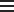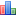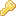## Item Identification

 Title: Climate_Vulnerability_Entities

## Entity Information

 Entity Type: Spreadsheet Yes Data dictionary for the climate vulnerability analysis data used in the "Coral Reef Resilience and Social Vulnerability to Climate Change" reports for the main Hawaiian Islands, Guam, the Commonwealth of the Northern Mariana Islands, and American Samoa.

## Data Attributes

### Attribute Summary

 Name Type Description100 Benthic_Sector TEXT Name of Spatial Reporting Sector. Contains the 3-letter island code (ISLANDCODE) or the island / atoll name (ISLAND). This is the field to join the data in this table with the SECTRNM field in the SECTOR shapefile, so the data can be plotted in a mapping application (e.g., ArcGIS). 100 IslandCode TEXT Three-letter code for ISLAND. 100 Island TEXT Name of island or atoll surveyed in the Pacific Islands region (REGIONCODE). 100 RegionCode TEXT The region surveyed in the Pacific Islands region: AMSM=American Samoa, PRIAs = Pacific Remote Island Areas, CNMI = Guam and the Commonwealth of the Northern Mariana Islands (Mariana Archipelago), MHI = main Hawaiian Islands, and NWHI = Northwestern Hawaiian Islands. 100 FAC_Den.Diseased NUMBER Raw data for Coral Disease: Observed number of diseased colonies per square meter. 100 FAC_CoralDiversity NUMBER Raw data for Coral Diversity: Diversity of hard corals. Expressed as the effective number of coral genera (exponential of the Shannon entropy index). 100 FAC_CoralJuvDensity NUMBER Raw data for Juvenile Coral Density: Observed mean number of corals under 5 cm per square meter. 100 FAC_HerbivorousFishBiomass NUMBER Raw data for Herbivorous Fish Biomass: Observed biomass of herbivorous fishes, grams per 100 meters square. 100 FAC_MacroalgalCover_FishTeam NUMBER Raw data for Macroalgal Cover: Observed mean percent cover of macroalgae. 100 FAC_BleachingResistance NUMBER Raw data for Bleaching Resistant Corals: Proportion of bleaching resistant coral species in an area, calculated from observed proportional abundance by species and observed patterns of bleaching by species. 100 FAC_FishingDepletion NUMBER Raw data for Fishing Depletion: Percent depletion of reef fishes due to fishing mortality, calculated from differences between observed biomass and biomass expected in the absence of fishing pressure (Williams et al. 2015). 100 FAC_TemperatureVariability NUMBER Raw data for Temperature Variability: History of high temperature variability in an area, calculated from the climatological summer temperature range divided by the annual temperature range (Heron et al. 2016). 100 N0595FAC_Den.Diseased NUMBER Normalized Coral Disease Metric (Observed number of diseased colonies per square meter.), normalized to be between 0 and 1 using the 5% quantile (q05) and 95% quantile (q95) of a sector's region according to Xnorm = (X - q05)/(q95-q05). The rare values below 0 or above 1 are set to 0 and 1 respectively. 100 N0595FAC_CoralDiversity NUMBER Normalized Coral Diversity Metric (Diversity of hard corals, expressed as the effective number of coral genera (exponential of the Shannon entropy index)), normalized to be between 0 and 1 using the 5% quantile (q05) and 95% quantile (q95) of a sector's region according to Xnorm = (X - q05)/(q95-q05). The rare values below 0 or above 1 are set to 0 and 1 respectively. 100 N0595FAC_CoralJuvDensity NUMBER Normalized Juvenile Coral Density Metric (Observed mean number of corals under 5 cm per square meter), normalized to be between 0 and 1 using the 5% quantile (q05) and 95% quantile (q95) of a sector's region according to Xnorm = (X - q05)/(q95-q05). The rare values below 0 or above 1 are set to 0 and 1 respectively. 100 N0595FAC_HerbivorousFishBiomass NUMBER Normalized Herbivorous Fish Biomass Metric (Observed biomass of herbivorous fishes, grams per 100 meters square), normalized to be between 0 and 1 using the 5% quantile (q05) and 95% quantile (q95) of a sector's region according to Xnorm = (X - q05)/(q95-q05). The rare values below 0 or above 1 are set to 0 and 1 respectively. 100 N0595FAC_MacroalgalCover_FishTeam NUMBER Normalized Macroalgal Cover Metric (Observed mean percent cover of macroalgae), normalized to be between 0 and 1 using the 5% quantile (q05) and 95% quantile (q95) of a sector's region according to Xnorm = (X - q05)/(q95-q05). The rare values below 0 or above 1 are set to 0 and 1 respectively. 100 N0595FAC_BleachingResistance NUMBER Normalized Bleaching Resistant Corals Metric (Proportion of bleaching resistant coral species in an area, calculated from observed proportional abundance by species and observed patterns of bleaching by species), normalized to be between 0 and 1 using the 5% quantile (q05) and 95% quantile (q95) of a sector's region according to Xnorm = (X - q05)/(q95-q05). The rare values below 0 or above 1 are set to 0 and 1 respectively. 100 N0595FAC_FishingDepletion NUMBER Normalized Fishing Depletion Metric (Percentage depletion of reef fishes due to fishing mortality, calculated from differences between observed biomass and biomass expected in the absence of fishing pressure (Williams et al. 2015)), normalized to be between 0 and 1 using the 5% quantile (q05) and 95% quantile (q95) according to Xnorm = (X - q05)/(q95-q05). The rare values below 0 or above 1 are set to 0 and 1 respectively. 100 N0595FAC_TemperatureVariability NUMBER Normalized Temperature Variability Metric (History of high temperature variability in an area, calculated from the climatological summer temperature range divided by the annual temperature range (Heron et al. 2016)), normalized to be between 0 and 1 using the 5% quantile (q05) and 95% quantile (q95) of a sector's region according to Xnorm = (X - q05)/(q95-q05). The rare values below 0 or above 1 are set to 0 and 1 respectively. 100 TotalResilience NUMBER Total Resilience Metric: Unweighted mean of all eight normalized factors. 100 TotalResilienceNorm NUMBER Normalized Total Resilience Metric, (unweighted mean of all eight normalized factors), normalized to be between 0 and 1 using the 5% quantile (q05) and 95% quantile (q95) of a sector's region according to Xnorm = (X - q05)/(q95-q05). The rare values below 0 or above 1 are set to 0 and 1 respectively. 100 Resistance NUMBER Bleaching Resistance: Unweighted mean of the normalized factors Bleaching Resistant Corals and Thermal Variability. 100 ResistanceNorm NUMBER Normalized Bleaching Resistance Metric: (unweighted mean of the normalized factors Bleaching Resistant Corals and Thermal Variability), normalized to be between 0 and 1 using the 5% quantile (q05) and 95% quantile (q95) of a sector's region according to Xnorm = (X - q05)/(q95-q05). The rare values below 0 or above 1 are set to 0 and 1 respectively. 100 CoralCompetitiveness NUMBER Coral Competitiveness: Unweighted mean of the normalized factors Coral Diversity, Herbivorous Fish Biomass, Macroalgal Cover, and Juvenile Coral Density. 100 CoralCompetitivenessNorm NUMBER Normalized Coral Competitiveness Metric: (unweighted mean of the normalized factors Coral Diversity, Herbivorous Fish Biomass, Macroalgal Cover, and Juvenile Coral Density), normalized to be between 0 and 1 using the 5% quantile (q05) and 95% quantile (q95) of a sector's region according to Xnorm = (X - q05)/(q95-q05). The rare values below 0 or above 1 are set to 0 and 1 respectively. 100 Stress.Free NUMBER Other Stressors: Unweighted mean of the normalized factors Fishing Depletion and Coral Disease. 100 Stress.FreeNorm NUMBER Normalized Other Stressors Metric (unweighted mean of the normalized factors Fishing Depletion and Coral Disease), normalized to be between 0 and 1 using the 5% quantile (q05) and 95% quantile (q95) of a sector's region according to Xnorm = (X - q05)/(q95-q05). The rare values below 0 or above 1 are set to 0 and 1 respectively.

### Benthic_Sector

CC ID: 909013
 Seq. Order: 1 TEXT No No Active Name of Spatial Reporting Sector. Contains the 3-letter island code (ISLANDCODE) or the island / atoll name (ISLAND). This is the field to join the data in this table with the SECTRNM field in the SECTOR shapefile, so the data can be plotted in a mapping application (e.g., ArcGIS).

### IslandCode

CC ID: 909014
 Seq. Order: 2 TEXT No No Active Three-letter code for ISLAND.

### Island

CC ID: 911295
 Seq. Order: 3 TEXT No No Active Name of island or atoll surveyed in the Pacific Islands region (REGIONCODE).

### RegionCode

CC ID: 909015
 Seq. Order: 4 TEXT No No Active The region surveyed in the Pacific Islands region: AMSM=American Samoa, PRIAs = Pacific Remote Island Areas, CNMI = Guam and the Commonwealth of the Northern Mariana Islands (Mariana Archipelago), MHI = main Hawaiian Islands, and NWHI = Northwestern Hawaiian Islands.

### FAC_Den.Diseased

CC ID: 909016
 Data Storage Type: NUMBER No No Active Raw data for Coral Disease: Observed number of diseased colonies per square meter.

### FAC_CoralDiversity

CC ID: 909017
 Data Storage Type: NUMBER No No Active Raw data for Coral Diversity: Diversity of hard corals. Expressed as the effective number of coral genera (exponential of the Shannon entropy index).

### FAC_CoralJuvDensity

CC ID: 909018
 Data Storage Type: NUMBER No No Active Raw data for Juvenile Coral Density: Observed mean number of corals under 5 cm per square meter.

### FAC_HerbivorousFishBiomass

CC ID: 909019
 Data Storage Type: NUMBER No No Active Raw data for Herbivorous Fish Biomass: Observed biomass of herbivorous fishes, grams per 100 meters square.

### FAC_MacroalgalCover_FishTeam

CC ID: 909020
 Data Storage Type: NUMBER No No Active Raw data for Macroalgal Cover: Observed mean percent cover of macroalgae.

### FAC_BleachingResistance

CC ID: 909021
 Data Storage Type: NUMBER No No Active Raw data for Bleaching Resistant Corals: Proportion of bleaching resistant coral species in an area, calculated from observed proportional abundance by species and observed patterns of bleaching by species.

### FAC_FishingDepletion

CC ID: 909022
 Data Storage Type: NUMBER No No Active Raw data for Fishing Depletion: Percent depletion of reef fishes due to fishing mortality, calculated from differences between observed biomass and biomass expected in the absence of fishing pressure (Williams et al. 2015).

### FAC_TemperatureVariability

CC ID: 909023
 Data Storage Type: NUMBER No No Active Raw data for Temperature Variability: History of high temperature variability in an area, calculated from the climatological summer temperature range divided by the annual temperature range (Heron et al. 2016).

### N0595FAC_Den.Diseased

CC ID: 909024
 Data Storage Type: NUMBER No No Active Normalized Coral Disease Metric (Observed number of diseased colonies per square meter.), normalized to be between 0 and 1 using the 5% quantile (q05) and 95% quantile (q95) of a sector's region according to Xnorm = (X - q05)/(q95-q05). The rare values below 0 or above 1 are set to 0 and 1 respectively.

### N0595FAC_CoralDiversity

CC ID: 909025
 Data Storage Type: NUMBER No No Active Normalized Coral Diversity Metric (Diversity of hard corals, expressed as the effective number of coral genera (exponential of the Shannon entropy index)), normalized to be between 0 and 1 using the 5% quantile (q05) and 95% quantile (q95) of a sector's region according to Xnorm = (X - q05)/(q95-q05). The rare values below 0 or above 1 are set to 0 and 1 respectively.

### N0595FAC_CoralJuvDensity

CC ID: 909026
 Data Storage Type: NUMBER No No Active Normalized Juvenile Coral Density Metric (Observed mean number of corals under 5 cm per square meter), normalized to be between 0 and 1 using the 5% quantile (q05) and 95% quantile (q95) of a sector's region according to Xnorm = (X - q05)/(q95-q05). The rare values below 0 or above 1 are set to 0 and 1 respectively.

### N0595FAC_HerbivorousFishBiomass

CC ID: 909027
 Data Storage Type: NUMBER No No Active Normalized Herbivorous Fish Biomass Metric (Observed biomass of herbivorous fishes, grams per 100 meters square), normalized to be between 0 and 1 using the 5% quantile (q05) and 95% quantile (q95) of a sector's region according to Xnorm = (X - q05)/(q95-q05). The rare values below 0 or above 1 are set to 0 and 1 respectively.

### N0595FAC_MacroalgalCover_FishTeam

CC ID: 909028
 Data Storage Type: NUMBER No No Active Normalized Macroalgal Cover Metric (Observed mean percent cover of macroalgae), normalized to be between 0 and 1 using the 5% quantile (q05) and 95% quantile (q95) of a sector's region according to Xnorm = (X - q05)/(q95-q05). The rare values below 0 or above 1 are set to 0 and 1 respectively.

### N0595FAC_BleachingResistance

CC ID: 909029
 Data Storage Type: NUMBER No No Active Normalized Bleaching Resistant Corals Metric (Proportion of bleaching resistant coral species in an area, calculated from observed proportional abundance by species and observed patterns of bleaching by species), normalized to be between 0 and 1 using the 5% quantile (q05) and 95% quantile (q95) of a sector's region according to Xnorm = (X - q05)/(q95-q05). The rare values below 0 or above 1 are set to 0 and 1 respectively.

### N0595FAC_FishingDepletion

CC ID: 909030
 Data Storage Type: NUMBER No No Active Normalized Fishing Depletion Metric (Percentage depletion of reef fishes due to fishing mortality, calculated from differences between observed biomass and biomass expected in the absence of fishing pressure (Williams et al. 2015)), normalized to be between 0 and 1 using the 5% quantile (q05) and 95% quantile (q95) according to Xnorm = (X - q05)/(q95-q05). The rare values below 0 or above 1 are set to 0 and 1 respectively.

### N0595FAC_TemperatureVariability

CC ID: 909031
 Data Storage Type: NUMBER No No Active Normalized Temperature Variability Metric (History of high temperature variability in an area, calculated from the climatological summer temperature range divided by the annual temperature range (Heron et al. 2016)), normalized to be between 0 and 1 using the 5% quantile (q05) and 95% quantile (q95) of a sector's region according to Xnorm = (X - q05)/(q95-q05). The rare values below 0 or above 1 are set to 0 and 1 respectively.

### TotalResilience

CC ID: 909032
 Data Storage Type: NUMBER No No Active Total Resilience Metric: Unweighted mean of all eight normalized factors.

### TotalResilienceNorm

CC ID: 909033
 Data Storage Type: NUMBER No No Active Normalized Total Resilience Metric, (unweighted mean of all eight normalized factors), normalized to be between 0 and 1 using the 5% quantile (q05) and 95% quantile (q95) of a sector's region according to Xnorm = (X - q05)/(q95-q05). The rare values below 0 or above 1 are set to 0 and 1 respectively.

### Resistance

CC ID: 909034
 Data Storage Type: NUMBER No No Active Bleaching Resistance: Unweighted mean of the normalized factors Bleaching Resistant Corals and Thermal Variability.

### ResistanceNorm

CC ID: 909035
 Data Storage Type: NUMBER No No Active Normalized Bleaching Resistance Metric: (unweighted mean of the normalized factors Bleaching Resistant Corals and Thermal Variability), normalized to be between 0 and 1 using the 5% quantile (q05) and 95% quantile (q95) of a sector's region according to Xnorm = (X - q05)/(q95-q05). The rare values below 0 or above 1 are set to 0 and 1 respectively.

### CoralCompetitiveness

CC ID: 909036
 Data Storage Type: NUMBER No No Active Coral Competitiveness: Unweighted mean of the normalized factors Coral Diversity, Herbivorous Fish Biomass, Macroalgal Cover, and Juvenile Coral Density.

### CoralCompetitivenessNorm

CC ID: 909037
 Data Storage Type: NUMBER No No Active Normalized Coral Competitiveness Metric: (unweighted mean of the normalized factors Coral Diversity, Herbivorous Fish Biomass, Macroalgal Cover, and Juvenile Coral Density), normalized to be between 0 and 1 using the 5% quantile (q05) and 95% quantile (q95) of a sector's region according to Xnorm = (X - q05)/(q95-q05). The rare values below 0 or above 1 are set to 0 and 1 respectively.

### Stress.Free

CC ID: 909038
 Data Storage Type: NUMBER No No Active Other Stressors: Unweighted mean of the normalized factors Fishing Depletion and Coral Disease.

### Stress.FreeNorm

CC ID: 909039
 Data Storage Type: NUMBER No No Active Normalized Other Stressors Metric (unweighted mean of the normalized factors Fishing Depletion and Coral Disease), normalized to be between 0 and 1 using the 5% quantile (q05) and 95% quantile (q95) of a sector's region according to Xnorm = (X - q05)/(q95-q05). The rare values below 0 or above 1 are set to 0 and 1 respectively.

## Catalog Details

 Catalog Item ID: 59260 gov.noaa.nmfs.inport:59260 Annette M DesRochers 2020-04-08 22:53+0000 SysAdmin InPortAdmin 2022-08-09 17:11+0000 2020-06-26 PIFSC Published Externally N 2020-06-26 1 Year 2021-06-26